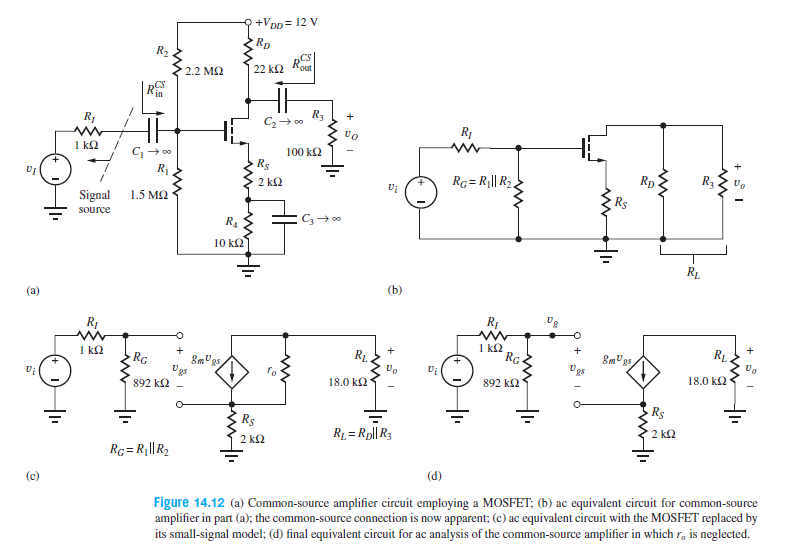# +Vpp= 12 V Rp R2 CS 22 k2 Kout 2.2 M2 | RS in R3 R, vo R1 1 k2 100 k2 Rs R1 Rc = R|| R2. Rp R3 2 kQ Signal 1.5 MQ Rs source R4 10 k2 RL (b) (a) R1 RI 1 k2 RG. 8mUgs gs R . 1 k2 R . RG gs 892 k2 18.0 k2 18.0 k2 892 k2 Rs Rs R1 = Rp|| R3 2 k2 2 k2 Rc = R||R2 Figure 14.12 (a) Common-source amplifier circuit employing a MOSFET; (b) ac equivalent circuit for common-source amplifier in part (a); the common-source connection is now apparent; (c) ac equivalent circuit with the MOSFET replaced by its small-signal model; (d) final equivalent circuit for ac analysis of the common-source amplifier in which r, is neglected.

Question

Calculate the Q-point for the transistor .help_outlineImage Transcriptionclose+Vpp= 12 V Rp R2 CS 22 k2 Kout 2.2 M2 | RS in R3 R, vo R1 1 k2 100 k2 Rs R1 Rc = R|| R2. Rp R3 2 kQ Signal 1.5 MQ Rs source R4 10 k2 RL (b) (a) R1 RI 1 k2 RG. 8mUgs gs R . 1 k2 R . RG gs 892 k2 18.0 k2 18.0 k2 892 k2 Rs Rs R1 = Rp|| R3 2 k2 2 k2 Rc = R||R2 Figure 14.12 (a) Common-source amplifier circuit employing a MOSFET; (b) ac equivalent circuit for common-source amplifier in part (a); the common-source connection is now apparent; (c) ac equivalent circuit with the MOSFET replaced by its small-signal model; (d) final equivalent circuit for ac analysis of the common-source amplifier in which r, is neglected. fullscreen

### Want to see this answer and more?

Experts are waiting 24/7 to provide step-by-step solutions in as fast as 30 minutes!*

*Response times may vary by subject and question complexity. Median response time is 34 minutes for paid subscribers and may be longer for promotional offers.
Tagged in
Engineering
Electrical Engineering

### Electrical Machines